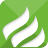﻿ DC-AC inverter: classification introduction-SCIENTEK ELECTRICAL

HOME NEWS DC-AC inverter: classification introduction

Sep 24,2020

# DC-AC inverter: classification introduction

The device that converts DC voltage to AC voltage is called a DC-AC converter or "inverter". The inverter converts the battery voltage to 110V AC or 220V AC. To save the electrical energy of household sockets, the development of AC-DC converters (rectifiers) appeared.

DC batteries can provide DC, and electrical equipment is designed to use AC power, which makes inverters appear in our lives. So what are the types of DC-AC inverters? What's the difference?DC-AC inverter: square wave inverter

This is the simplest inverter. The power supply voltage is Vs. Consider the RL load. When Q1 and Q2 conduct, the output is connected to +Vs. When Q3 and Q4 conduct, the output is connected to –Vs. The AC output voltage is not the same as the sine wave but has some AC characteristics, which may be useful in some applications.

The current flowing through the switch must be bidirectional, but true switches, such as insulated gate bipolar transistors (IGBTs), can only flow in one direction. So we added anti-parallel feedback diodes to each switch. During the interval when the switching current should be negative, the diode will conduct current. In a positive cycle, the diode will be reverse biased.

DC-AC inverter: full bridge inverter

This inverter is composed of four switches connected by a bridge. By properly opening and closing two switches at a time, a 2-level AC (pulsating DC) output can be obtained. According to the different states of the switch, the output level will fluctuate between +VDC and –VDC. A real switch requires switching transition time, which is called blanking time.

DC-AC inverter: multi-level inverter

The output shape of these types of inverters is very similar to sine waves, and they have a wide range of applications, including speed-regulated motor drive and the connection of renewable energy sources such as photovoltaics to the grid.

In a dual-source inverter, one voltage source and H-bridge must output longer pulses than the other voltage source and H-bridge to obtain a sine wave output. In this way, one DC power supply (battery) will discharge faster than the other. A technique called mode switching is used for each DC power battery for the same time on average. The first source must perform a longer period in the first half of the cycle, and the other source must perform a longer period in the second half of the cycle. In this way, during a complete cycle, the behavior of the two sources is equal.

DC-AC inverter: Diode clamped multi-level inverter

Multi-level inverter circuit with multiple DC voltage source batteries. We can use multiple capacitors and only one DC power battery. The capacitors will be connected in series and use a single DC battery as the power source. The output voltage level of the dual-capacitor single-cell multi-level inverter is (VDC, VDC/2, 0, -VDC/2, -VDC).

0.181652s